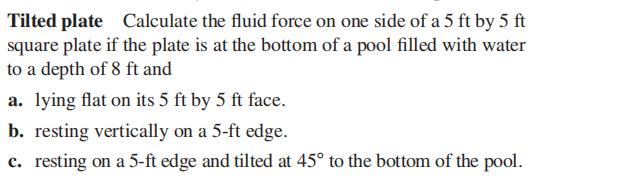### Still have math questions?

Trigonometry
QuestionTilted plate Calculate the fluid force on one side of a $$5 ft$$ by $$5 ft$$ square plate if the plate is at the bottom of a pool filled with water to a depth of $$8 ft$$ and

a. lying flat on its $$5 ft$$ by $$5 ft$$ face.

b. resting vertically on a $$5$$ -ft edge.

c. resting on a $$5$$ -ft edge and tilted at $$45 ^ { \circ }$$ to the bottom of the pool.

$$\left. \begin{array} { l l l l } { \text { (a) } 12,480 lb } & { \text { (b) } 8580 lb } & { \text { (c) } 9722.3 lb } \end{array} \right.$$